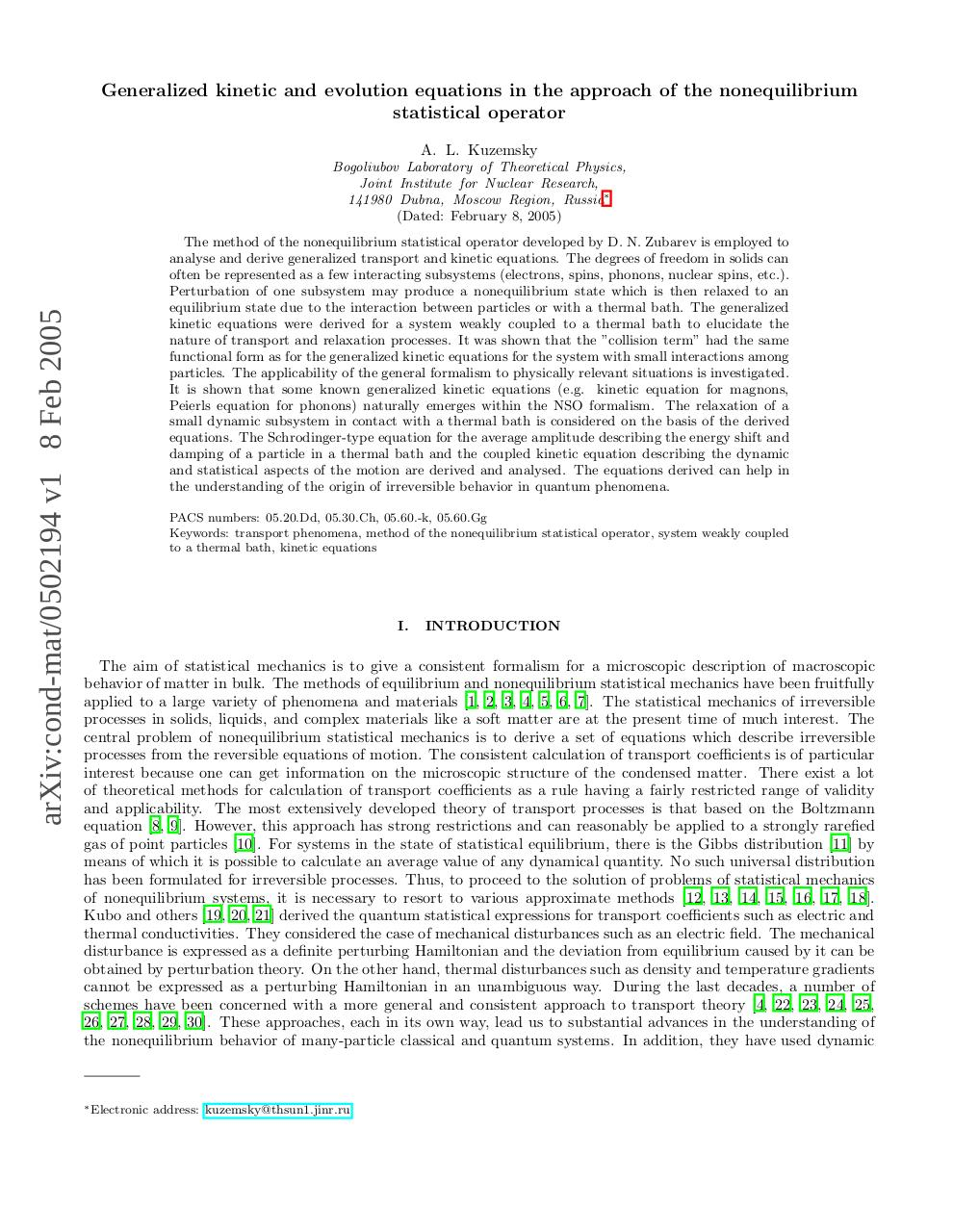PDF Archive

Easily share your PDF documents with your contacts, on the Web and Social Networks.

Nonequilibrium Statistical Operator IJMPB05.pdfPage 12321

Text preview

Generalized kinetic and evolution equations in the approach of the nonequilibrium
statistical operator
A. L. Kuzemsky

arXiv:cond-mat/0502194 v1 8 Feb 2005

Bogoliubov Laboratory of Theoretical Physics,
Joint Institute for Nuclear Research,
141980 Dubna, Moscow Region, Russia∗
(Dated: February 8, 2005)
The method of the nonequilibrium statistical operator developed by D. N. Zubarev is employed to
analyse and derive generalized transport and kinetic equations. The degrees of freedom in solids can
often be represented as a few interacting subsystems (electrons, spins, phonons, nuclear spins, etc.).
Perturbation of one subsystem may produce a nonequilibrium state which is then relaxed to an
equilibrium state due to the interaction between particles or with a thermal bath. The generalized
kinetic equations were derived for a system weakly coupled to a thermal bath to elucidate the
nature of transport and relaxation processes. It was shown that the ”collision term” had the same
functional form as for the generalized kinetic equations for the system with small interactions among
particles. The applicability of the general formalism to physically relevant situations is investigated.
It is shown that some known generalized kinetic equations (e.g. kinetic equation for magnons,
Peierls equation for phonons) naturally emerges within the NSO formalism. The relaxation of a
small dynamic subsystem in contact with a thermal bath is considered on the basis of the derived
equations. The Schrodinger-type equation for the average amplitude describing the energy shift and
damping of a particle in a thermal bath and the coupled kinetic equation describing the dynamic
and statistical aspects of the motion are derived and analysed. The equations derived can help in
the understanding of the origin of irreversible behavior in quantum phenomena.
PACS numbers: 05.20.Dd, 05.30.Ch, 05.60.-k, 05.60.Gg
Keywords: transport phenomena, method of the nonequilibrium statistical operator, system weakly coupled
to a thermal bath, kinetic equations

I.

INTRODUCTION

The aim of statistical mechanics is to give a consistent formalism for a microscopic description of macroscopic
behavior of matter in bulk. The methods of equilibrium and nonequilibrium statistical mechanics have been fruitfully
applied to a large variety of phenomena and materials [1, 2, 3, 4, 5, 6, 7]. The statistical mechanics of irreversible
processes in solids, liquids, and complex materials like a soft matter are at the present time of much interest. The
central problem of nonequilibrium statistical mechanics is to derive a set of equations which describe irreversible
processes from the reversible equations of motion. The consistent calculation of transport coefficients is of particular
interest because one can get information on the microscopic structure of the condensed matter. There exist a lot
of theoretical methods for calculation of transport coefficients as a rule having a fairly restricted range of validity
and applicability. The most extensively developed theory of transport processes is that based on the Boltzmann
equation [8, 9]. However, this approach has strong restrictions and can reasonably be applied to a strongly rarefied
gas of point particles . For systems in the state of statistical equilibrium, there is the Gibbs distribution  by
means of which it is possible to calculate an average value of any dynamical quantity. No such universal distribution
has been formulated for irreversible processes. Thus, to proceed to the solution of problems of statistical mechanics
of nonequilibrium systems, it is necessary to resort to various approximate methods [12, 13, 14, 15, 16, 17, 18].
Kubo and others [19, 20, 21] derived the quantum statistical expressions for transport coefficients such as electric and
thermal conductivities. They considered the case of mechanical disturbances such as an electric field. The mechanical
disturbance is expressed as a definite perturbing Hamiltonian and the deviation from equilibrium caused by it can be
obtained by perturbation theory. On the other hand, thermal disturbances such as density and temperature gradients
cannot be expressed as a perturbing Hamiltonian in an unambiguous way. During the last decades, a number of
schemes have been concerned with a more general and consistent approach to transport theory [4, 22, 23, 24, 25,
26, 27, 28, 29, 30]. These approaches, each in its own way, lead us to substantial advances in the understanding of
the nonequilibrium behavior of many-particle classical and quantum systems. In addition, they have used dynamic

∗ Electronic